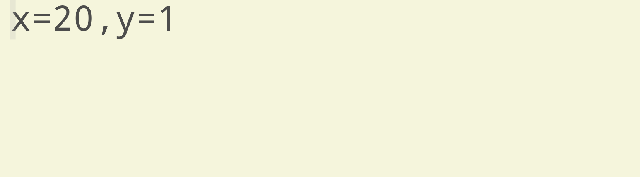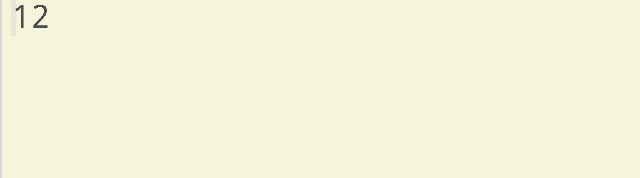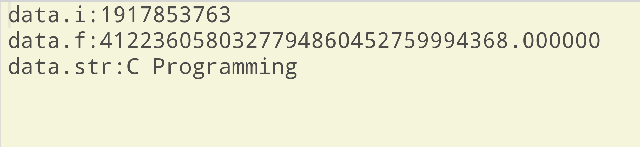# Structure and Union in C

Previously, we learnt about pointers in C, but in this article, we are going to know about structures and union in C. Arrays allow us to define the type of variable that can hold several data items of the same kind. Likewise, structure is another user defined data type available in C that allows to combine data items of different kinds.Structure and Union in C

## Definition of structure

A structure is a user defined data type in C that lets us combine data items of different kinds.

Structures are used to represent a record. Assume you want to keep track of your books in a library. You can keep track of those books with the following data:

• Title
• Author
• Subject
• Book ID

## How to create a structure

struct’ keyword is used to create a structure. For example:

``````struct details
{
char title;
char author;
char subject;
char bookid;
float price;
};``````

## Declaration of structure variables

A structure variable can either be declared with structure declaration or as a separate declaration like fundamental types.

``````/*A variable declaration with structure declaration.*/
struct Point
{
int x,y;
}p1;  /*The variable p1 is declared with 'Point'*/

/*A variable declaration like basic data types*/
struct Point
{
int x,y;
};
int main()
{
struct Point p1;  /*The variable p1 is declared like a normal variable*/
}``````

## Initialising structure members

Structure members cannot be initialized with declaration. For example the following C program fails in compilation.

``````struct Point
{
int x=0;  /*COMPILER ERROR: cannot initialize members here*/
int y=0;  /*COMPILER ERROR: cannot initialize members here*/
};``````

The reason for above error is simple, when a datatype is declared but no memory is allocated for it. Memory is allocated only when variables are created.

Structure members are initialized using curly braces ‘{}’. For example, following is a valid initialization.

``````struct Point
{
int x,y;

};
int main()
{
/*A valid initialization. Member x gets value 0 and y gets value 1. The order of declaration is followed.*/
struct Point p1={0,1};
}``````

## Accessing structure elements

Structure members are accessed using dot (.) operator.

``````#include<stdio.h>
struct Point
{
int x,y;
};
int main()
{
struct Point p1={0,1};
/*Accesing members of point p1*/
p1.x=20;
printf ("x=%d,y=%d",p1.x,p1.y);
return 0;
}``````

#### Output:Accessing structure elements

## Array of structures

Like other primitive data types, we can create an array of structures.

``````#include<stdio.h>
struct Point
{
int x, y;
};
int main()
{
/*Create an array of structures*/
struct Point arr;
/*Access array members*/
arr.x=10;
arr.y=20;
printf("%d%d",arr.x,arr.y);
return 0;
}``````

#### Output:Array of Structures

## Definition of structure pointer

If we have a pointer to structure, members are accessed using the arrow ( -> ) operator.

``````#include<stdio.h>
struct Point
{
int x,y;
};
int main()
{
struct Point p1={1,2};
/*p2 is a pointer to structure p1*/
struct Point *p2=&p1;
/*Accessing structure members using structure pointer*/
printf("%d%d",p2->x,p2->y);
return 0;
}``````

#### Output:Output Structure

## Definition of union

To define a union, one must use the union statement in the same way as we did while defining a structure. The union statement defines a new data type with more than one member for a program. The syntax of the union statement is as follows:

``````union [union tag] {
member definition;
member definition;
...
member definition;
} [one or more union variables];``````

The union tag is optional and each member definition is the usual variable definition, such as int a; or float b; or any other valid variable definition. Before ending the union definition, it is optional, but you can add one/more union variables. Here is the way to define a union type named Data having three members a, b, and str:

``````union Data{
int i;
float f;
char str;
}data;``````

Now, a variable of Data type can store either an integer, or, a floating point number, or may be a string of characters. It means a single variable, i.e., same memory location, can now be used to store several types of data. One can use any built-in or user defined data type inside a union based on his/her necessity.

The memory that is occupied by a union will be large enough to contain the largest member of the union. Like in the above example, Data type will occupy 20 bytes of space from memory as this is the maximum space that can be occupied by a character string.

## Accessing union members

In order to access any member of a union, we use a member access operator (.). That operator is coded as a period between the union variable name and the union member that we wish to access. You have to use the keyword union to define variables that are of union type. The following example will explain how to use unions in a C program:

``````#include<stdio.h>
#include<string.h>
union Data{
int i;
float f;
char str;
};
int main()
{
union Data data;
data.i=10;
data.f=220.5;
strcpy(data.str,"C Programming");
printf("data.i:%d\n",data.i);
printf("data.f:%f\n",data.f);
printf("data.str:%s\n",data.str);
return 0;
}``````

#### Output:Accessing Union Members

## Pointer to Union

Like structures, we can let pointers access to unions and the members can be accessed using the arrow operator (->). Study the example below to understand.

``````#include<stdio.h>
union test
{
int x;
char y;
};
int main()
{
union test p1;
p1.x = 65;
/*p2 is a pointer to union p1*/
union test* p2 = &p1;
/*Accessing union members using pointer*/
printf("%d %c", p2->x, p2->y);
return 0;
}``````

#### Output:Output Union in C

All programs written in this post are compiled online.

### Books I Prefer:I hope this article was helpful to understand structure and union in C! Comment below, if you've got any question. Head back soon for another interesting article on C Programming.